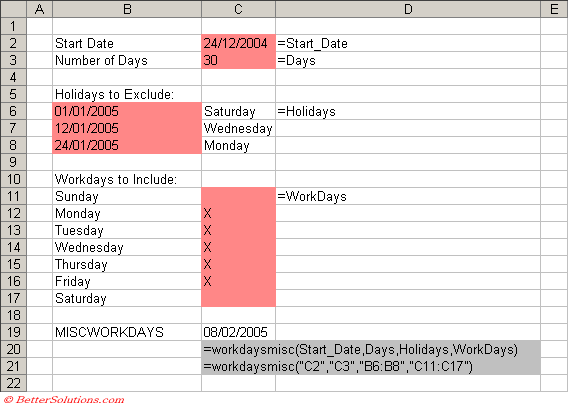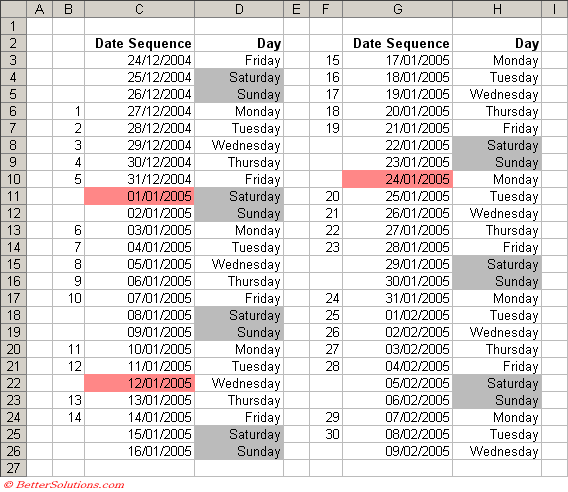# WORKDAYSMISC

Returns the serial number before or after a given number of days from a start date using a defined list of workdays.
For instructions on how to add a function to a workbook refer to the page under Inserting Functions

`Option Base 1    'lStartDate - The starting date.'lDays - The number of valid days after the begin date that the event should take place.'rgeHolidays - The dates to exclude from the working calendar, holidays and floating days.'rgeWorkdays - The days of the week that you want to include.Public Function WORKDAYSMISC(ByVal lStartDate As Long, _                              ByVal lDays As Long, _                              ByVal rgeHolidays As Range, _                              ByVal rgeWorkDays As Range) As Long  Dim iweekdaycount As Integer Dim bhasvalidworkday As Boolean Dim bisvalidworkday As Boolean Dim lnewdate As Long Dim idaycount As Integer Dim arholidays() As Long Dim iholidaycount As Integer Dim iarrayno As Integer    bhasvalidworkday = False    For iweekdaycount = 1 To 7       If rgeWorkDays.Item(iweekdaycount).Text <> "" Then bhasvalidworkday = True       If rgeWorkDays.Item(iweekdaycount).Text <> "" Then Exit For    Next iweekdaycount       If bhasvalidworkday = False Then Call MsgBox("The rgeWorkDays parameter is incorrect")    If bhasvalidworkday = False Then Exit Function       ReDim arholidays(rgeHolidays.Count)    For iholidaycount = 1 To rgeHolidays.Count       If rgeHolidays.Item(iholidaycount).Value <> "" Then          arholidays(iholidaycount) = rgeHolidays.Item(iholidaycount).Value       Else          Exit For       End If    Next iholidaycount    ReDim Preserve arholidays(iholidaycount - 1)       lnewdate = lStartDate    idaycount = lDays       Do Until idaycount = 0       bisvalidworkday = True          If lDays = 0 Then idaycount = 1       If lDays > 0 Then lnewdate = lnewdate + 1       If lDays < 0 Then lnewdate = lnewdate - 1          If rgeWorkDays.Item(VBA.Weekday(lnewdate)).Text <> "" Then          For iarrayno = 1 To UBound(arholidays)             If lnewdate = arholidays(iarrayno) Then                bisvalidworkday = False                Exit For             End If          Next iarrayno             If bisvalidworkday = True Then             If idaycount > 0 Then idaycount = idaycount - 1             If idaycount < 0 Then idaycount = idaycount + 1          End If       End If    Loop    WORKDAYSMISC = lnewdate End Function `

This user defined function is similar to the built-in WORKDAY function.